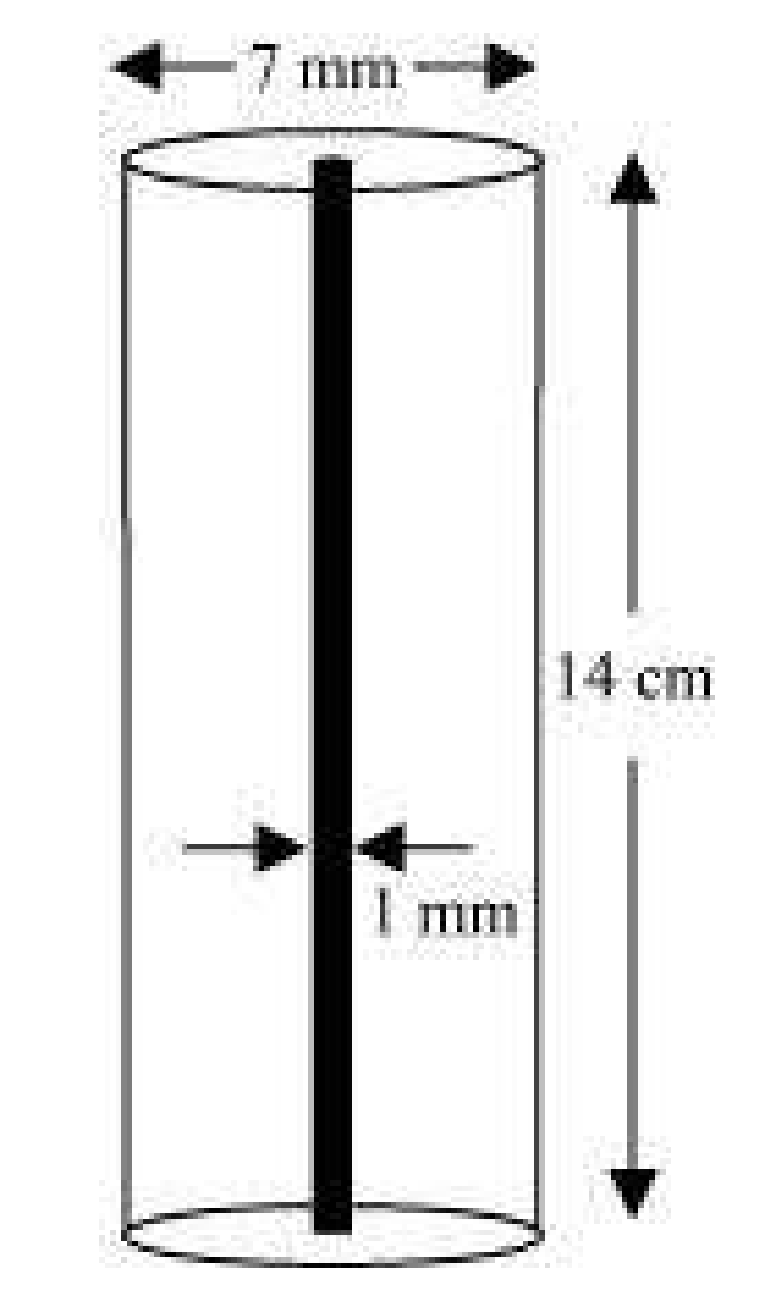# A lead pencil consists of a cylinder of wood with solid cylinder of graphite filled in the interior. The diameter of the pencil is 7 mm and the diameter of the graphite is 1 mm. If the length of the pencil is 14 cm, find the volume of the wood and that of the graphite. (Assume π = 22/7)Given

Height of wood (and graphite) cylinder (h) = 14 cm = 140 mm

Find out

We have to find out the volume of the wood and that of the graphite

Solution

Volume of Graphite = (πr2h)

On taking π=22/7

= 22/7 X 1/2 X 1/2× 140 mm3

= 110 mm3

= 0.11 cm3

Volume of wood = Volume of pencil – Volume of graphite

On taking π=22/7

(πR2h) – (πr2h) = π(R2 – r2)h

=22/7 [(7/2)2 -(1/2)2 x 140 mm3

= 22 × 20 × (49/4-1/4) mm3

= 22 × 20 × 12 mm3

= 5280 mm3

= 52.80cm3